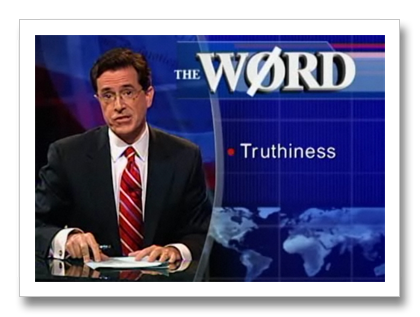## Truthiness

Computers have a very strict idea of when things are true and false.(Unlike Stephen Colbert...)

## True or False?

Try the following in irb:

• `1 < 2`
• `2 + 2 < 4`
• `2 + 2 <= 4`
• `2.even?`
• `4.odd?`
• `"apple".empty?`
• `"".empty?`

## Conditions

The magic word `if` is called a CONDITIONAL.

``````if age < 18 then
puts "Sorry, adults only."
end
``````

## One-Line Condition

Ruby has a compact way of putting an entire `if` expression on one line:

``````puts "Sorry, adults only." if age < 18
``````

Note that:

• the action comes first in a one-line condition
• this sounds kind of natural
• "Go to bed if you're sleepy."

## if... then... else... end

The magic word `else` allows BRANCHING.

``````if age >= 18 then
puts "allowed"
else
puts "denied"
end
``````

Like a fork in the road, the program chooses one path or the other.

(In Ruby, `then` is optional, so we usually leave it off, but if it makes your code clearer, go ahead and use it.)

## 2 + 2 = 4

``````2 + 2 = 4
``````

causes a `SyntaxError`. You need to do

``````2 + 2 == 4
``````

## The Tragedy of the Equal Sign

• a single equal sign means ASSIGNMENT
• `name = "Alice"` -- "assign the variable 'name' to the value 'Alice'"
• two equal signs means COMPARISON
• `name == "Alice"` -- "does the variable 'name' contain the string 'Alice'?"

This is confusing, and you should feel confused.

• (it's all FORTRAN's fault)

## LAB: Good Friend, Bad Friend

• Your `hello.rb` program should currently look something like this:
``````puts "What is your name?"
name = gets.strip
puts "Hello, " + name + "!"
``````
• Now change `hello.rb` so that it doesn't always say hello!
• If the user's name is "Darth" then say "Go away!"

## Conjunction Junction

• You can make more complicated logical expressions using conjunctions like `and`, `or`, `not`:

• `X and Y` means "are both X and Y true?"
• `X or Y` means "is either X or Y (or both) true?"
• `not X` means "is X false?"
• For example:

``````    if age >= 18 or parent.gave_permission? then
puts "allowed"
else
puts "denied"
end
``````

## LAB: Enemies List

• Change `hello.rb` so that it says "Go away!" if the user's name is any one of a number of evil names
• For instance, Voldemort, Satan, Lex Luthor...